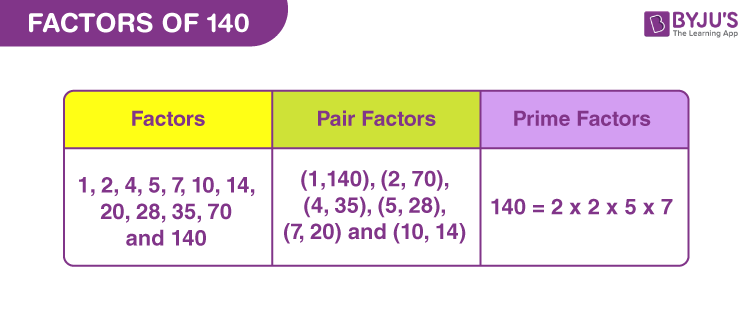# Factors of 140

Factors of 140 are the whole numbers that divide the original number, evenly or in equal parts. 140 is a composite number, thus it has more than two factors. When 140 is divided by its factor, then the quotient will be a natural number and the remainder is zero.

140 divided by 2 → 140 ÷ 2 = 70 (Remainder = 0)

In the same way, we can find the other factors of 140 here in this article. Let us find the pair factors and prime factors of 140.## How to Find the Factors of 140?

Factors of 140 are the integers that divide the original number, completely. To find the factors, we have to check with such numbers, that can divide 140 in equal parts. As we know, all the numbers are divisible by 1, therefore, 1 is one of the factors. Also, 140 is an even number, (unit place is 0), thus it is divisible by 2 and 5. Let us find more such factors.

• 140 ÷ 1 = 140
• 140 ÷ 2 = 70
• 140 ÷ 4 = 35
• 140 ÷ 5 = 28
• 140 ÷ 7 = 20
• 140 ÷ 10 = 14
• 140 ÷ 14 = 10
• 140 ÷ 20 = 7
• 140 ÷ 28 = 5
• 140 ÷ 35 = 4
• 140 ÷ 70 = 2
• 140 ÷ 140 = 1

Therefore, the required factors are: 1, 2, 4, 5, 7, 10, 14, 20, 28, 35, 70 and 140.

## Pair Factors of 140

To find the pair factors of 140, we need to find the product of the two numbers that result in the original number.

• 1 × 140 = 140
• 2 × 70 = 140
• 4 × 35 = 140
• 5 x 28 = 140
• 7 × 20 = 140
• 10 × 14 = 140

Therefore, the pair factors are (1,140), (2, 70), (4, 35) (5, 28), (7, 20) and (10, 14).

### Negative Pair Factors of 140

• -1 × -140 = 140
• -2 × -70 = 140
• -4 × -35 = 140
• -5 × -28 = 140
• -7 × -20 = 140
• -10 × -14 = 140

Therefore, the negative pair factors are (-1,-140), (-2, -70), (-4, -35) (-5, -28), (-7, -20) and (-10, -14).

## Prime Factorisation of 140

By prime factorisation of 140, we can find the prime factors. In this case, we need to divide 140, starting with the smallest prime number (by which it is divisible), till we get 1.

Step 1: Dividing 140 by the smallest prime factor, which is 2, we get;

140/2 = 70

Step 2: Again we can divide 70 by 2.

70/2 = 35

Step 3: 35 is divisible by 5. Thus,

35/5 = 7

Step 4: 7 is itself a prime number. Thus,

7/7 = 1

Therefore,

 Prime factorisation of 140 = 2 x 2 x 5 x 7

## Solved Examples

Q.1: Seema bought 5 books for Rs. 140. What is the cost of each book?

Solution: Given,

Number of books = 5

Price of all the books = Rs.140

Cost of each book = Rs.140/5 = 28.

Therefore, each book cost Rs. 28.

Q.2: What is the sum of all the factors of 140?

Solution: The factors of 140 are 1, 2, 4, 5, 7, 10, 14, 20, 28, 35, 70 and 140.

Sum = 1 + 2 + 4 + 5 + 7 + 10 + 14 + 20 + 28 + 35 + 70 + 140 = 336

Therefore, 336 is the required sum.

Q.3: What are the common factors of 144 and 140?

Answer: Let us list down the factors of 140 and 144.

140 → 1, 2, 4, 5, 7, 10, 14, 20, 28, 35, 70, 140

144 → 1, 2, 3, 4, 6, 8, 9, 12, 16, 18, 24, 36, 48, 72, 144

Therefore, the common factors of 140 and 144 are 1, 2 and 4.

## Frequently Asked Questions on Factors of 140

### How many factors of 140 are there?

There are twelve factors of 140. They are 1, 2, 4, 5, 7, 10, 14, 20, 28, 35, 70 and 140.

### Is 140 a perfect square?

No, 140 is not a perfect square. Square root of 140 is an irrational number and not a whole number.

### What are the multiples of 140?

The first 10 multiples of 140 are 140, 280, 420, 560, 700, 840, 980, 1120, 1260, 1400.

### What product of prime numbers gives 140?

The prime factorisation of 140 is 2 x 2 x 5 x 7 or 22 x 5 x 7.

### What is the greatest factor of 140?

The greatest factor of 140 is 140.

### What is the GCF of 140 and 100?

The factors of 100: 1, 2, 4, 5, 10, 20, 25, 50, 100

Factors of 140: 1, 2, 4, 5, 7, 10, 14, 20, 28, 35, 70, 140.

Therefore, GCF (140, 100) = 20